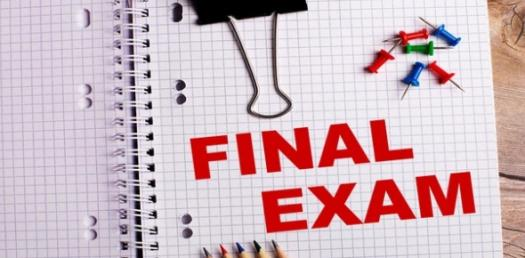# Semester Exam (2nd Period)

10 Questions | Total Attempts: 33SettingsSemester Exam for Video Program Design

• 1.
Negation Logical Negation p T _____ F T 
• A.

True

• B.

False

• 2.
Logical Conjunction p q p . q T T 1.____ T F 2.____ F T 3.____ F F 4.____
• A.

1. T 2. F 3. T 4. T

• B.

1. F 2. T 4. T 5. F

• C.

1. T 2. T 4. T 5. F

• D.

1. T 2. F 3. F 4. F

• 3.
Logical Disjunction p q p + q T T T F F T F F
• A.

1. T 2. F 3. F 4. F

• B.

1. T 2. T 3. T 4. F

• C.

1. F 2. T 4. T 5. F

• D.

1. T 2. F 3. T 4. T

• 4.
This symbol = OR which is a logical disjunction.
• A.

True

• B.

False

• 5.
This symbol = OR wihich is a logical disjunction.
• A.

True

• B.

False

• 6.
Commutative Law  A + B = A B =
• A.

B + A and B * A

• B.

(B + A) A and (B * A) A

• 7.
Associate Law (A + B) + C =  (A B) C =
• A.

(C + B + A) C and B( A + C)

• B.

A + (B + C) and A (B C)

• 8.
Distributive Law A (B + C) = A + (B C) =
• A.

A C + A A and A + B + B + C

• B.

A B + A C and (A + B) (A + C)

• 9.
Identity Law A + A = A A =
• A.

B and A

• B.

A and B

• C.

A and A

• 10.
(a) 0 + A = (b) 0 A =
• A.

0 and A

• B.

A and A

• C.

0 and 0

• D.

A and 0

Related TopicsBack to top